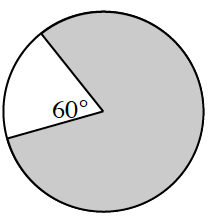### Home > GC > Chapter 8 > Lesson 8.3.1 > Problem8-90

8-90.

Multiple Choice: What fraction of the circle below is shaded?

1. $\frac { 60 } { 360 }$

1. $\frac { 300 } { 360 }$

1. $\frac { 60 } { 180 }$

1. $\frac { 120 } { 180 }$

1. None of these(b), because the portion of the shaded circle is $300º$ of the total $360º$.